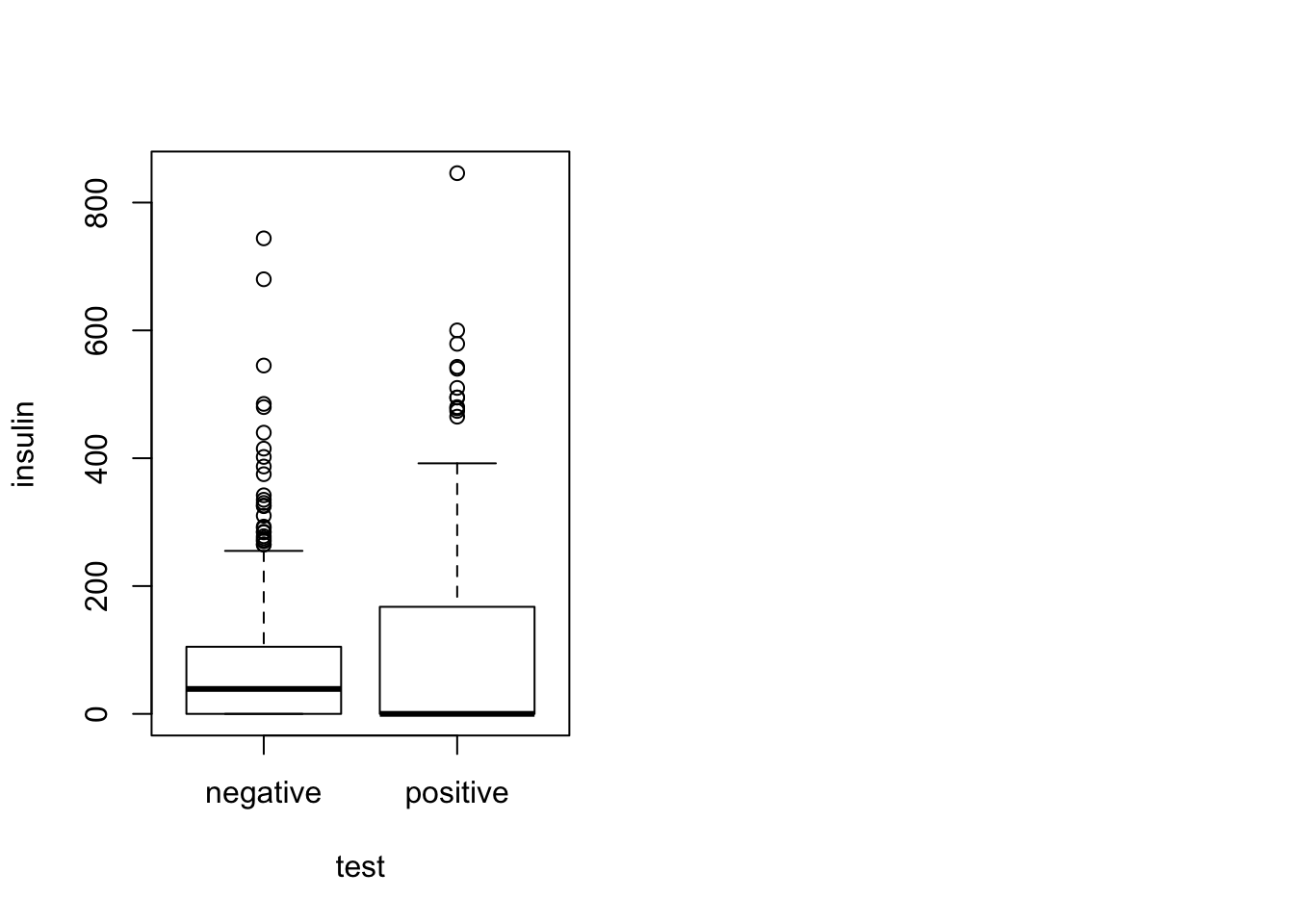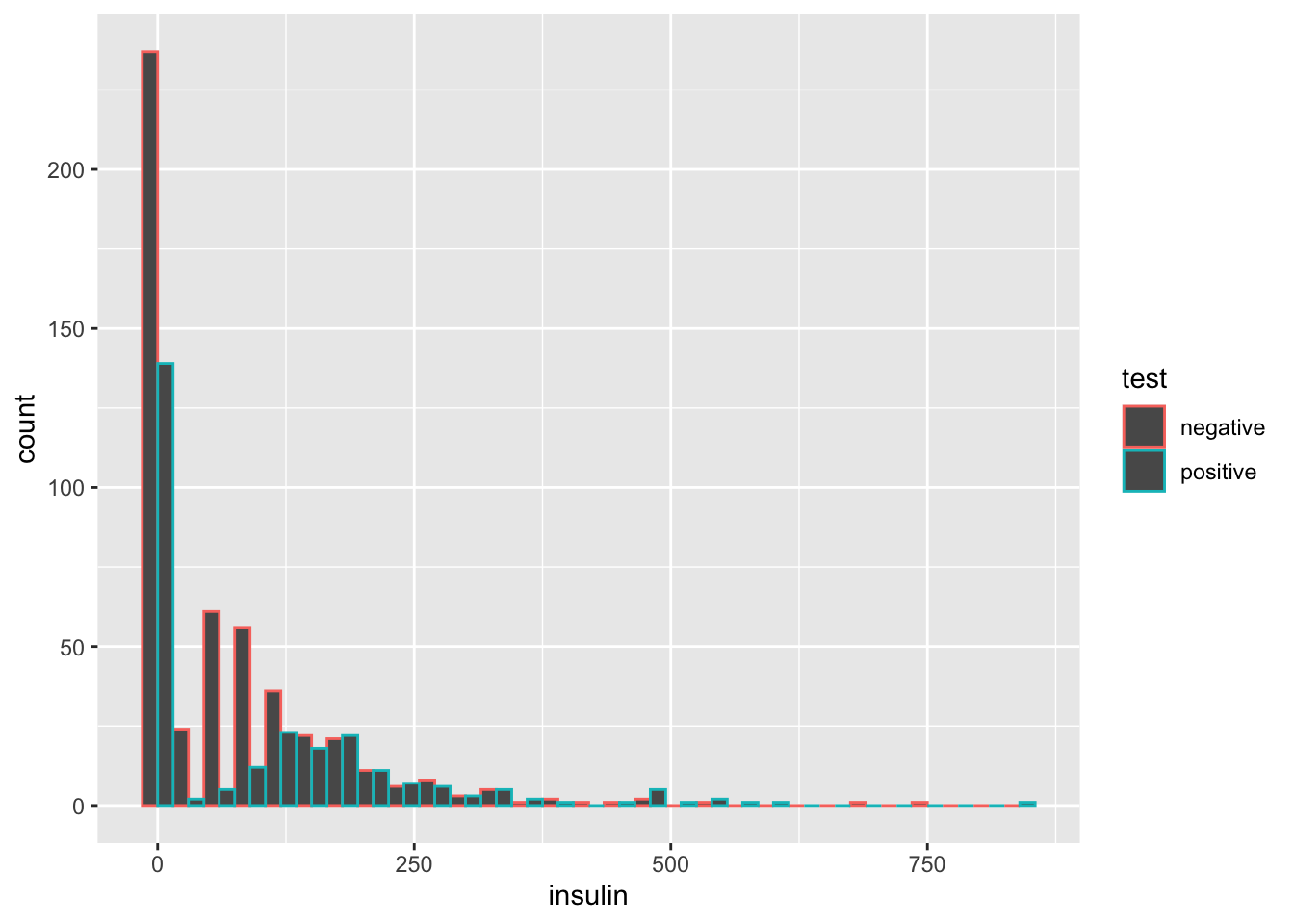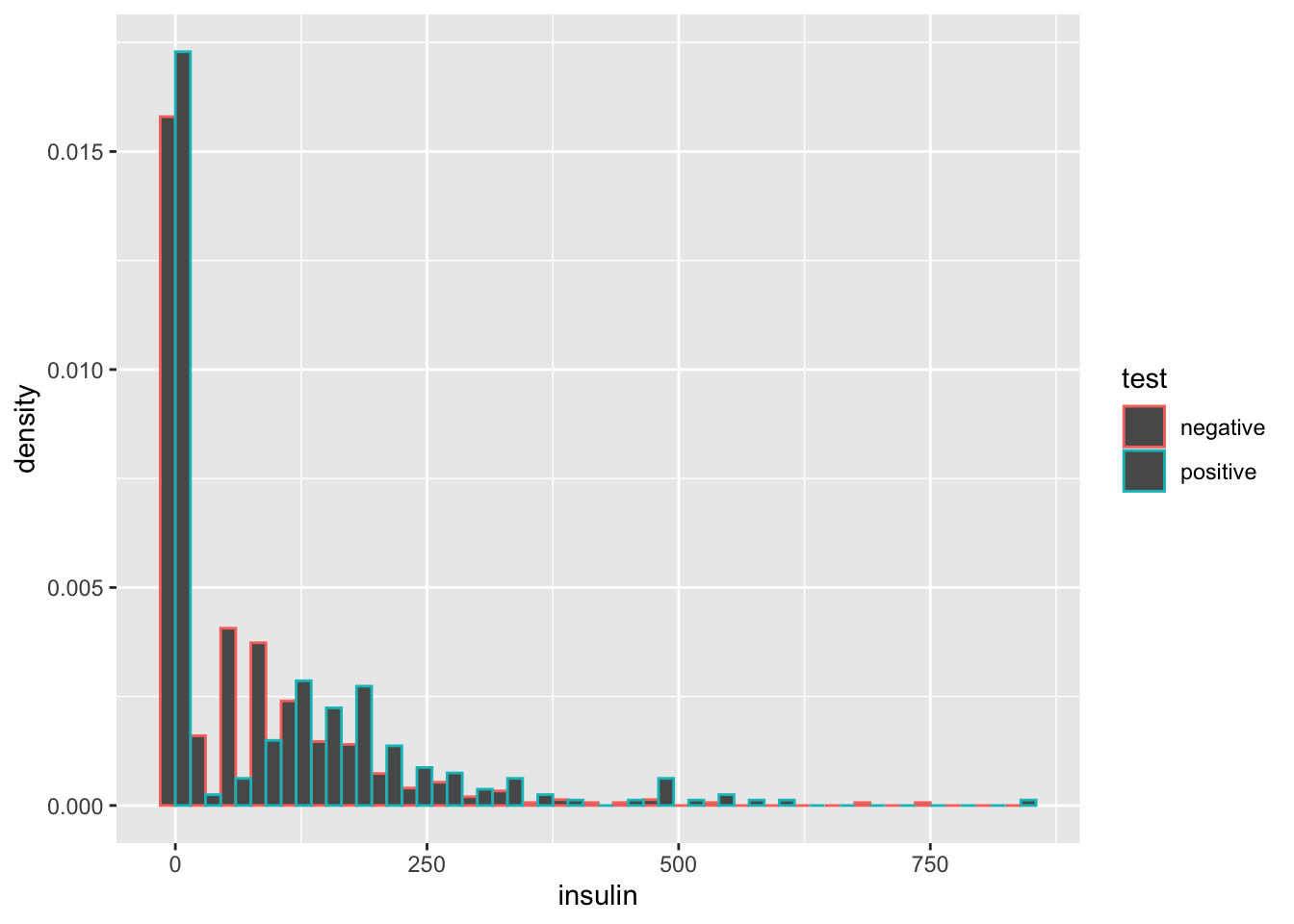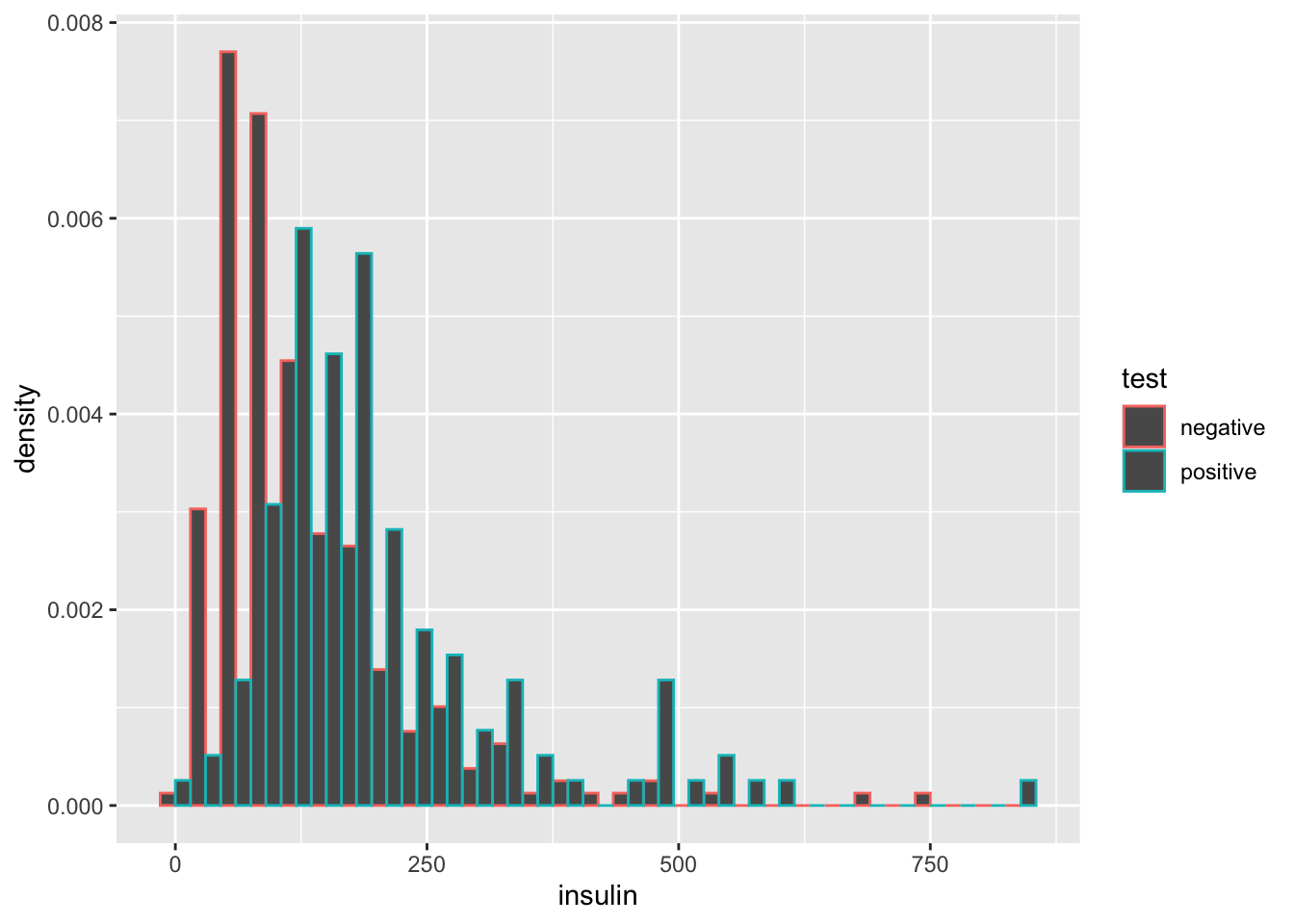# Analysis of Pima Dataset in R

Published:

In this blog post I would like to provide an anylsis of the Pima dataset that is available in the faraway R-package. The exercises below are part of the course MAST90139: Statistical Modelling for Data Science at the University of Melbourne. Analysis of Pima dataset in R (Diabetes survey on Pima Indians)

First we clean up any variables that we may have left in the environment

rm(list = ls())

# Load data and perform EDA

library(faraway)
library(ggplot2)
data(pima)
head(pima)
help(pima)
dim(pima)
##  768   9

## Exercise 1:

Create a factor version of the test results and use this to produce an interleaved histogram to show how the distribution of insulin differs between those testing positive and negative. Do you notice anything unbelievable about the plot?

pima$test = as.factor(pima$test)
levels(pima$test) <- c("negative","positive"); pima[1,] par(mfrow=c(1,2)); plot(insulin ~ test, pima)ggplot(pima, aes(x=insulin, color=test)) + geom_histogram(position="dodge", binwidth=30)library(ggplot2) ggplot(pima, aes(x = insulin, color = test)) + geom_histogram(position="dodge", binwidth=30, aes(y=..density..))summary(pima$test[pima$insulin==0]) ## negative positive ## 236 138 High values of insulin seem to correlate with signs of diabetes! ## Exercise 2: Replace the zero values of insulin with the missing value code NA. Recreate the interleaved histogram plot and comment on the distribution. pima$insulin[pima\$insulin == 0] <- NA
ggplot(pima, aes(x = insulin, color = test)) + geom_histogram(position="dodge",
binwidth=30, aes(y=..density..))
## Warning: Removed 374 rows containing non-finite values (stat_bin).After replacing the zero values with NA, the relationship becomes even more clearer!

## Exercise 3:

Replace the incredible zeroes in other variables with the missing value code. Fit a model with the result of the diabetes test as the response and all the other variables as predictors. How many observations were used in the model fitting? Why is this less than the number of observations in the data frame?

pima[pima == 0] <- NA
# Fit logistic regression model from binomial family
model1 <- glm(test ~ pregnant + glucose + diastolic + triceps + insulin + bmi + diabetes + age,family =  binomial, pima)
summary(model1)
##
## Call:
## glm(formula = test ~ pregnant + glucose + diastolic + triceps +
##     insulin + bmi + diabetes + age, family = binomial, data = pima)
##
## Deviance Residuals:
##     Min       1Q   Median       3Q      Max
## -2.8619  -0.6557  -0.3295   0.6158   2.6339
##
## Coefficients:
##               Estimate Std. Error z value Pr(>|z|)
## (Intercept) -1.083e+01  1.423e+00  -7.610 2.73e-14 ***
## pregnant     7.364e-02  5.973e-02   1.233   0.2176
## glucose      3.616e-02  6.249e-03   5.785 7.23e-09 ***
## diastolic    5.993e-03  1.320e-02   0.454   0.6497
## triceps      1.110e-02  1.869e-02   0.594   0.5527
## insulin      3.231e-05  1.445e-03   0.022   0.9822
## bmi          7.615e-02  3.174e-02   2.399   0.0164 *
## diabetes     1.097e+00  4.777e-01   2.297   0.0216 *
## age          4.075e-02  1.919e-02   2.123   0.0337 *
## ---
## Signif. codes:  0 '***' 0.001 '**' 0.01 '*' 0.05 '.' 0.1 ' ' 1
##
## (Dispersion parameter for binomial family taken to be 1)
##
##     Null deviance: 426.34  on 335  degrees of freedom
## Residual deviance: 288.92  on 327  degrees of freedom
##   (432 observations deleted due to missingness)
## AIC: 306.92
##
## Number of Fisher Scoring iterations: 5

In the pima dataframe, there are 768 observations, but only 327+9=336 observations were used to fit the model. 432 observations were deleted due to missingness.

plot(model1)